【图像压缩】基于matlab BP神经网络图像压缩【含Matlab源码 741期】

一、简介

1 概述
BP（Back Propagation）神经网络是1986年由Rumelhart和McCelland为首的科研小组提出，参见他们发表在Nature上的论文 Learning representations by back-propagating errors 。

BP神经网络是一种按误差逆传播算法训练的多层前馈网络，是目前应用最广泛的神经网络模型之一。BP网络能学习和存贮大量的 输入-输出模式映射关系，而无需事前揭示描述这种映射关系的数学方程。它的学习规则是使用最速下降法，通过反向传播来不断 调整网络的权值和阈值，使网络的误差平方和最小。

2 BP算法的基本思想

BP算法的信号流向图如下图所示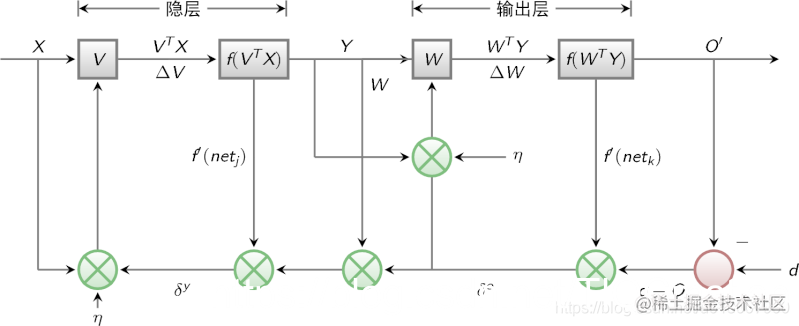3 BP网络特性分析——BP三要素

1)网络拓扑结构；
2)传递函数；
3)学习算法。3.1 BP网络的拓扑结构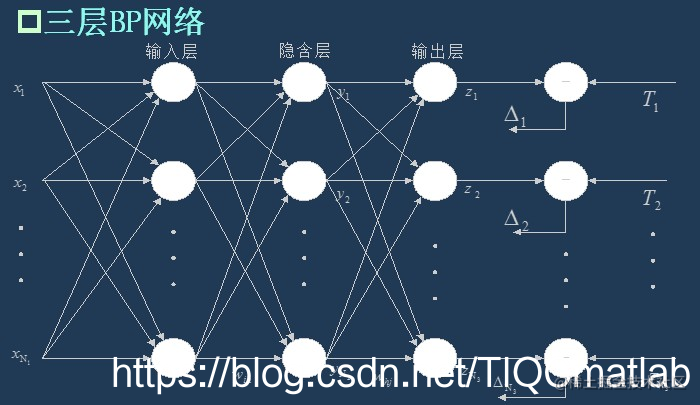3.2 BP网络的传递函数
BP网络采用的传递函数是非线性变换函数——Sigmoid函数（又称S函数）。其特点是函数本身及其导数都是连续的，因而在处理上十分方便。为什么要选择这个函数，等下在介绍BP网络的学习算法的时候会进行进一步的介绍。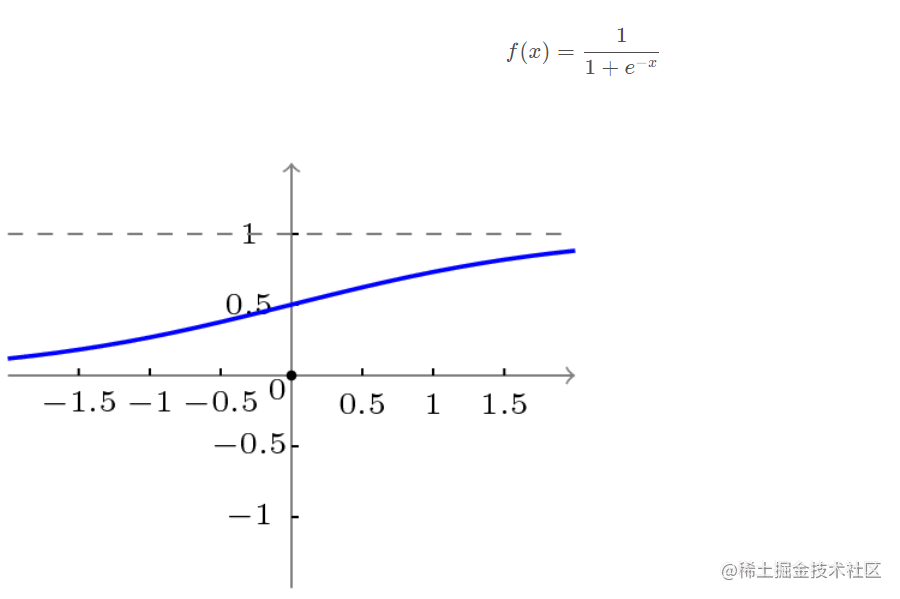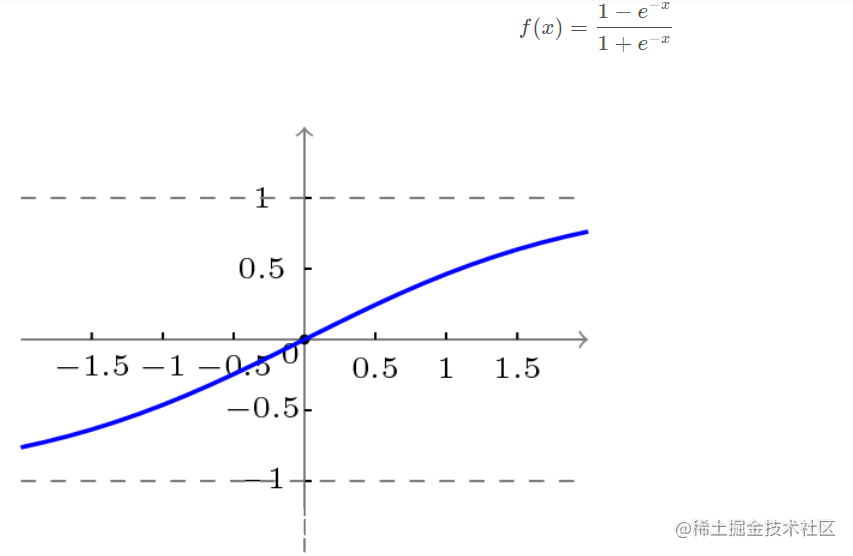3.3 BP网络的学习算法
BP网络的学习算法就是BP算法，又叫 δ 算法（在ANN的学习过程中我们会发现不少具有多个名称的术语）， 以三层感知器为例，当网络输出与期望输出不等时，存在输出误差 E ，定义如下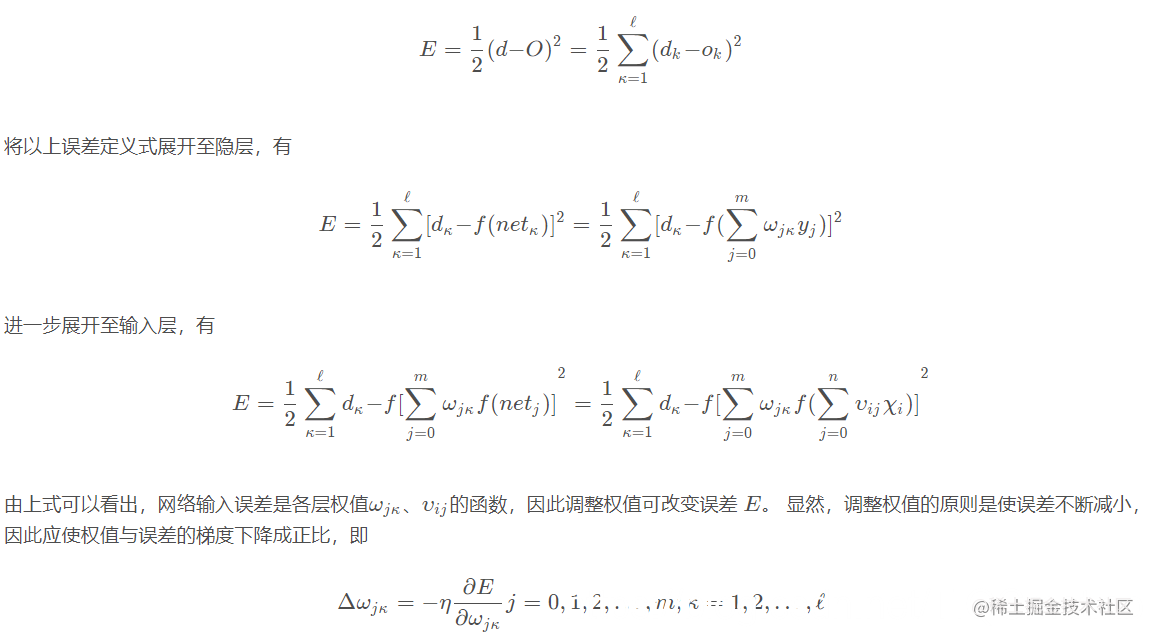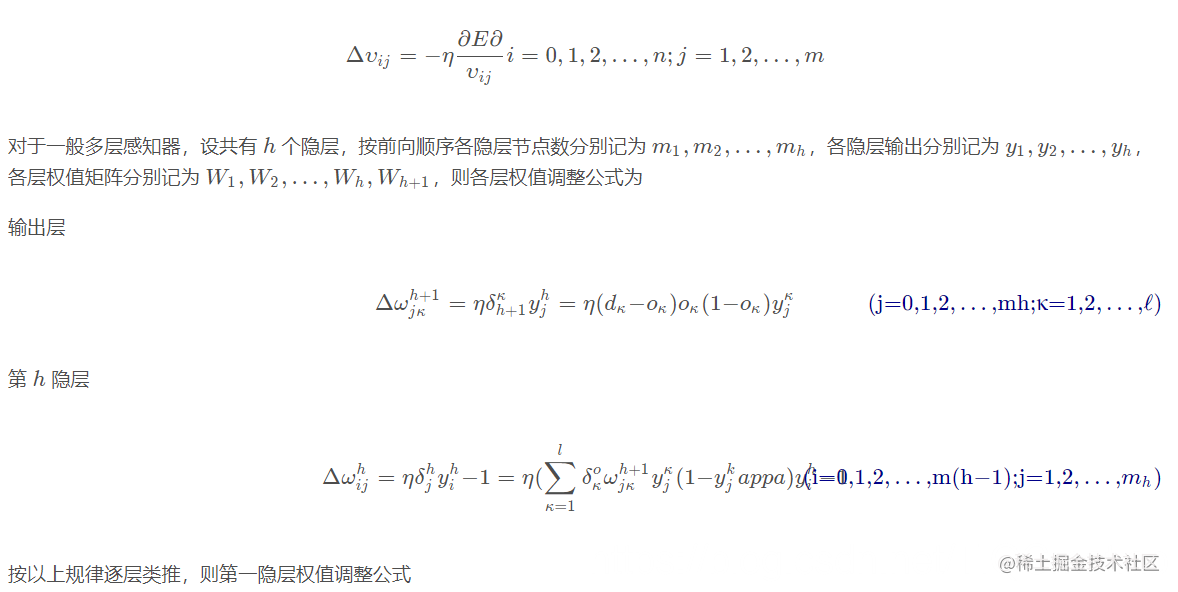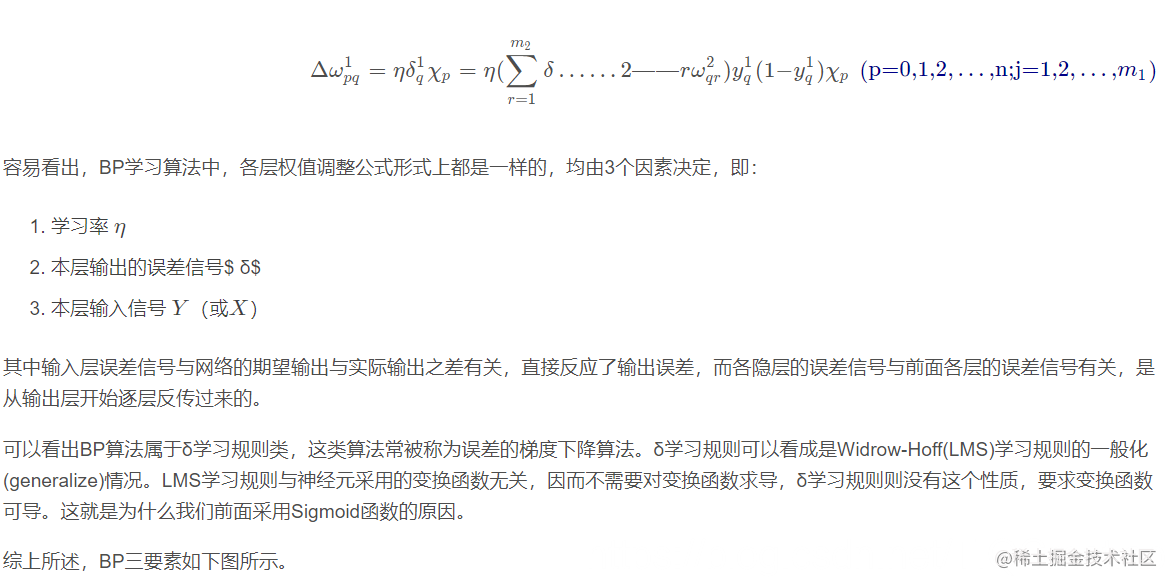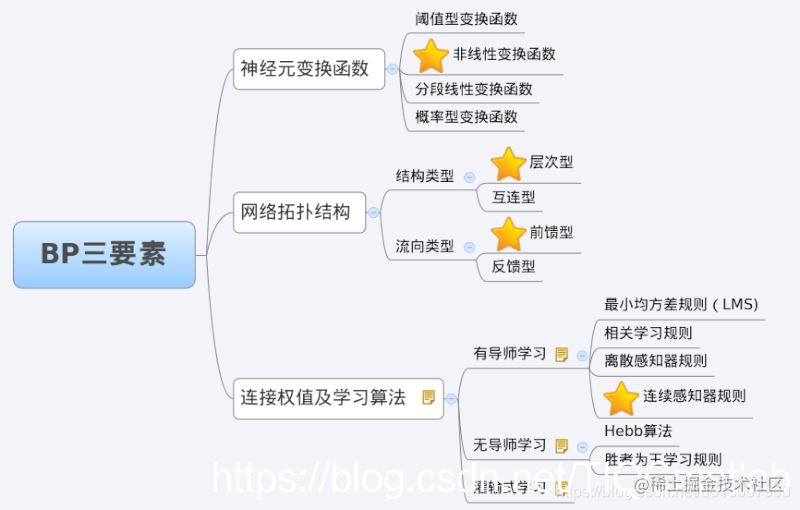4 BP网络的训练分解

while 终止条件未满足：
for record:dataset:
trainModel(record)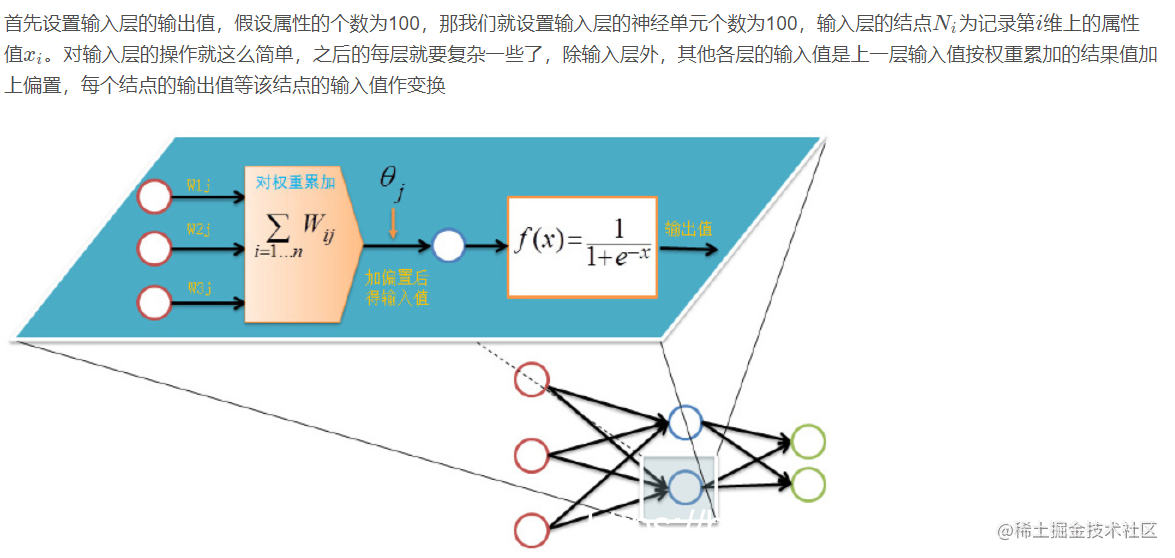4.1 逆向反馈（Backpropagation）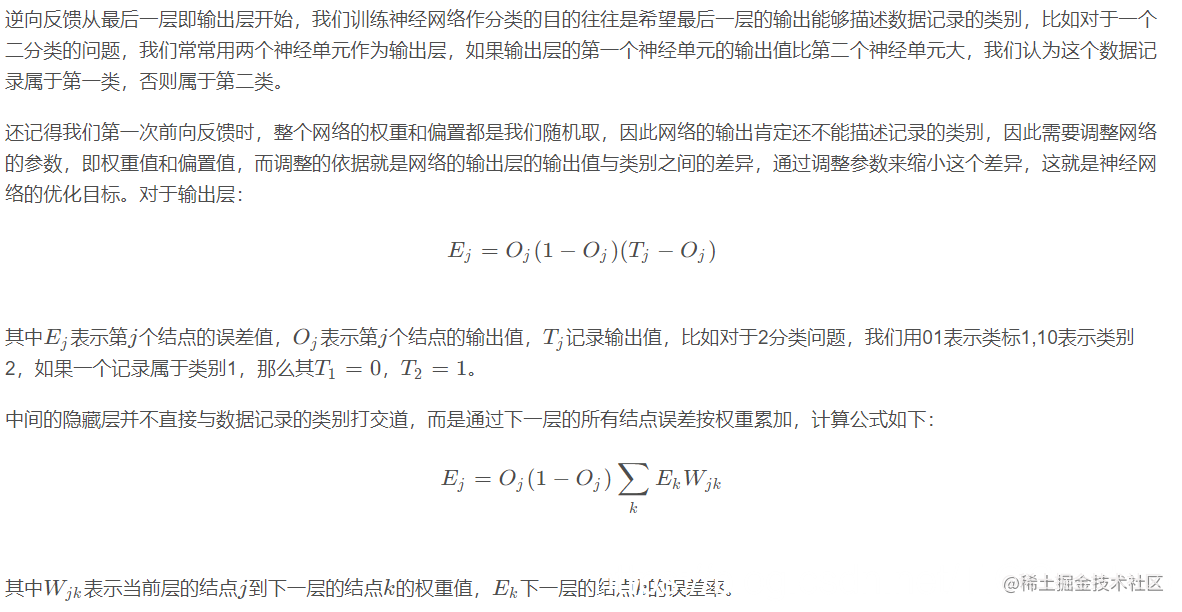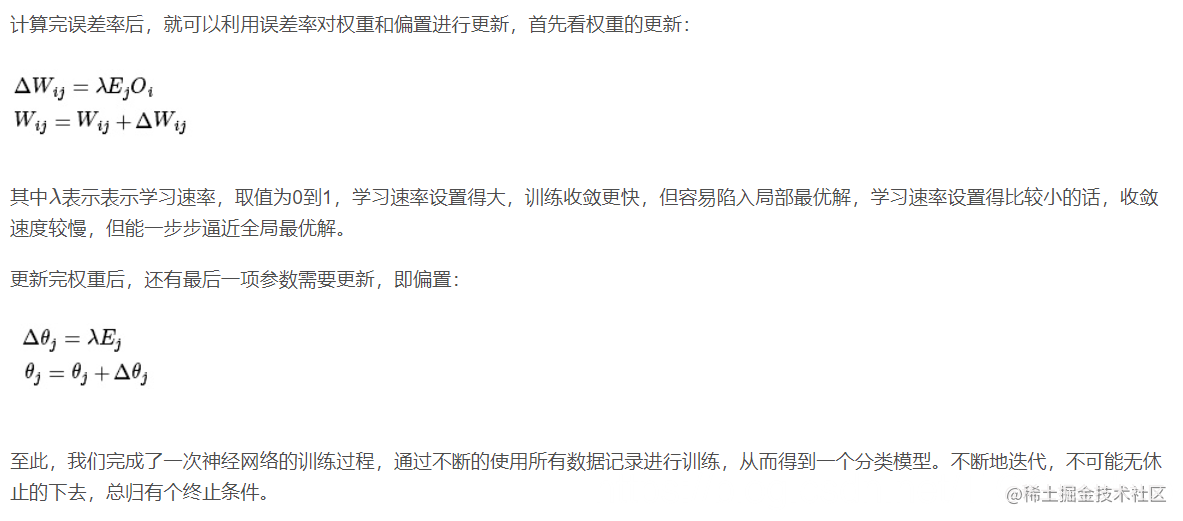4.2 训练终止条件

5 BP网络运行的具体流程
5.1 网络结构

5.2 变量定义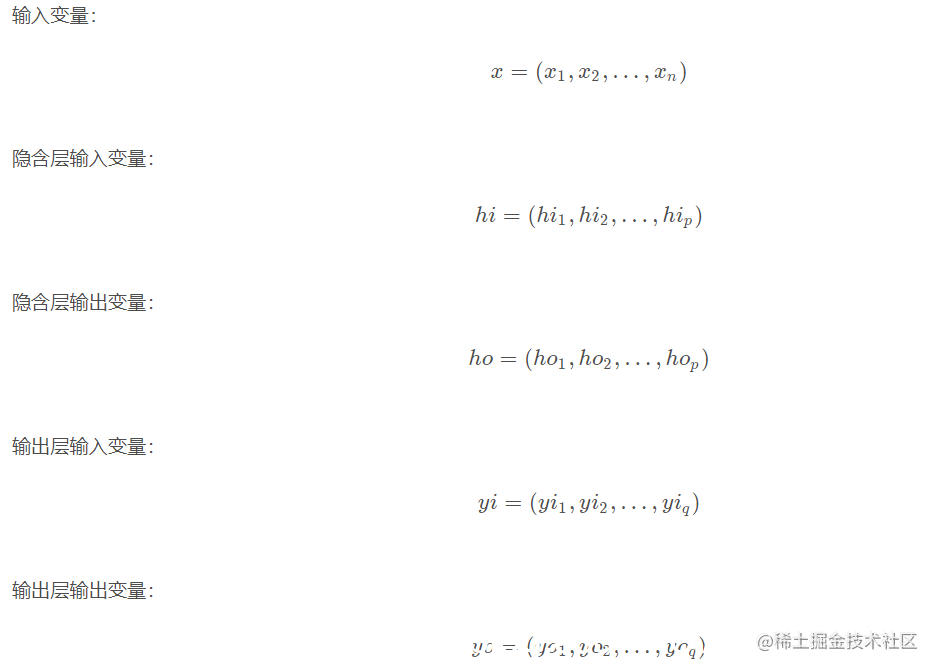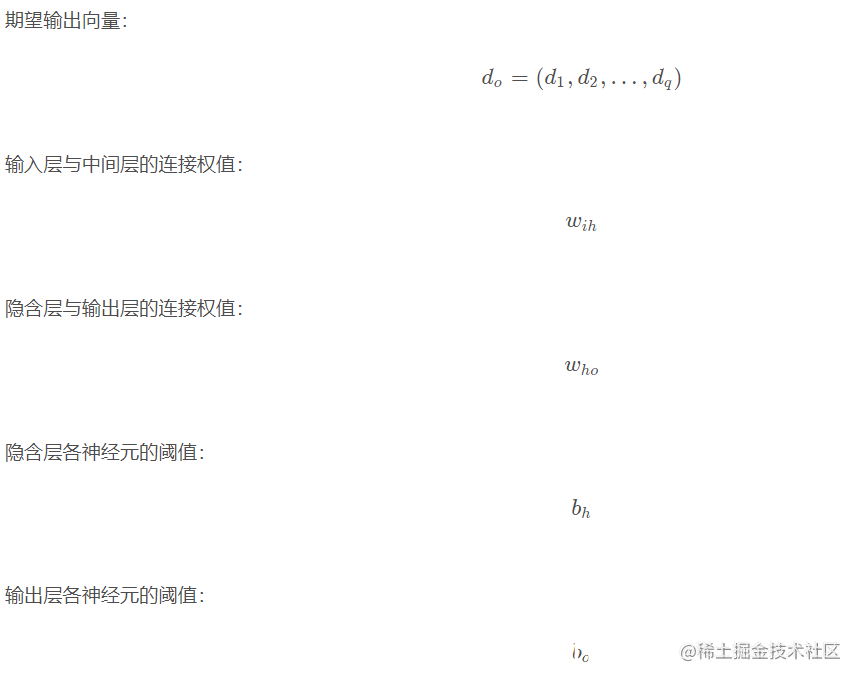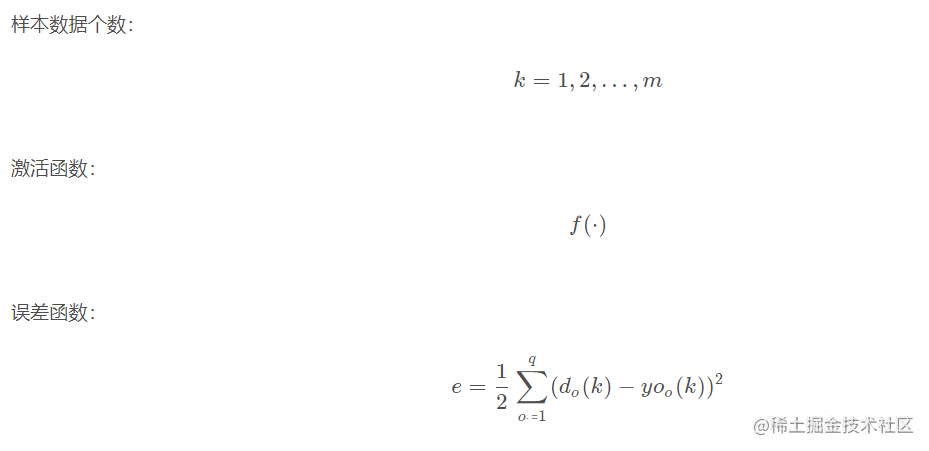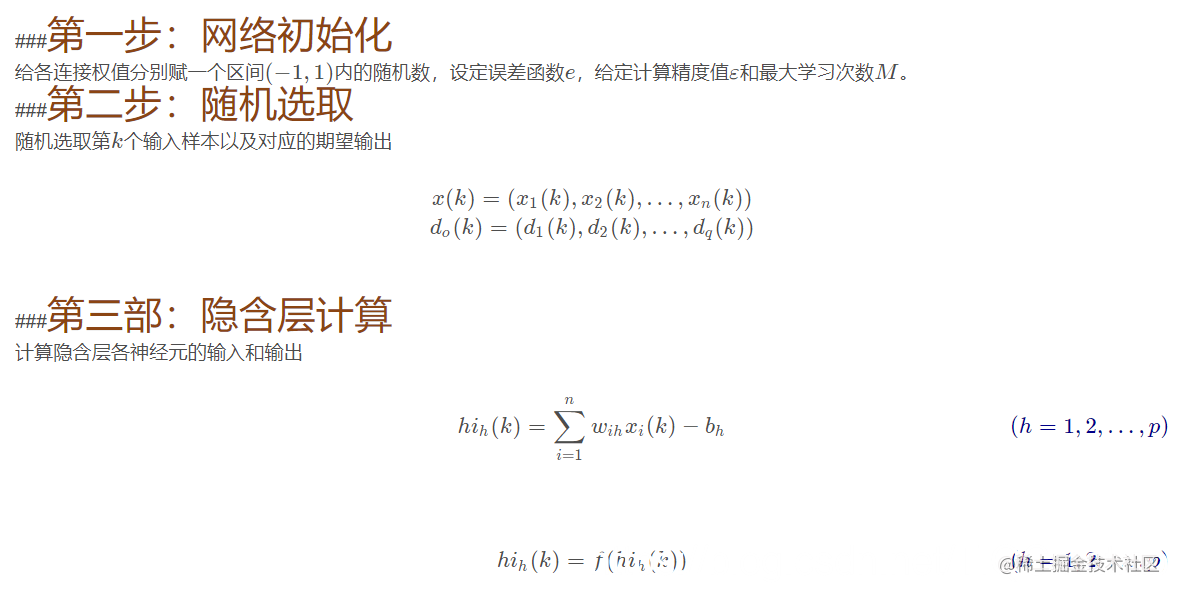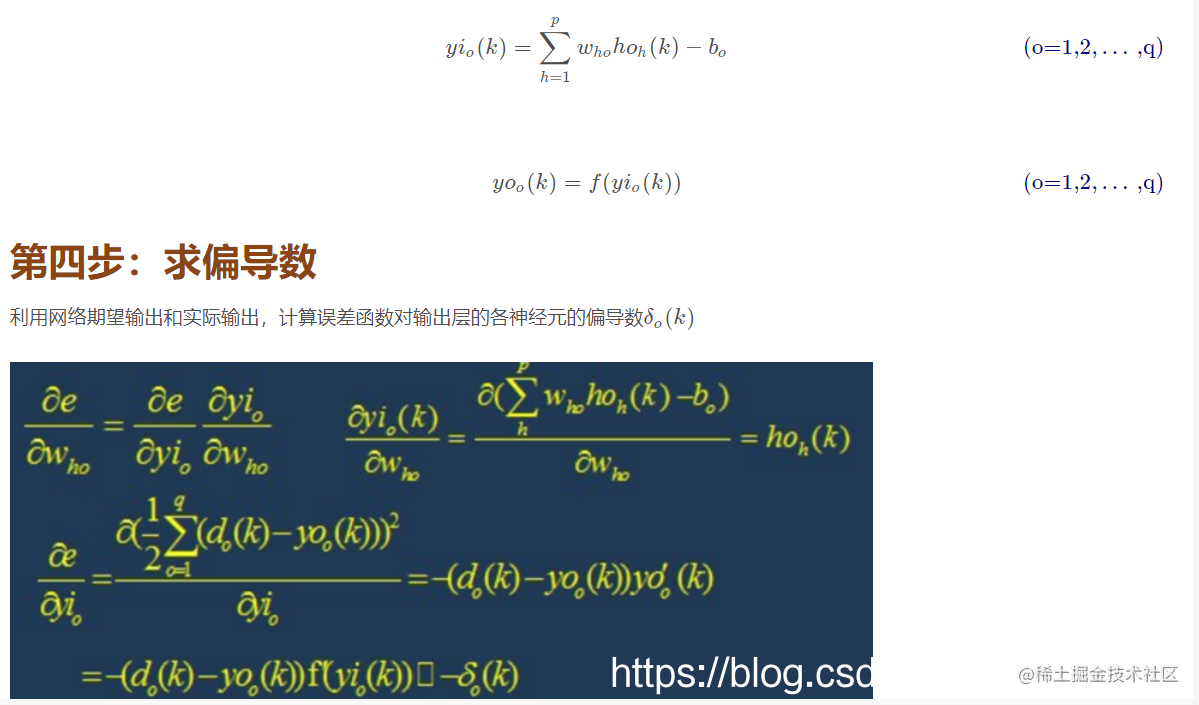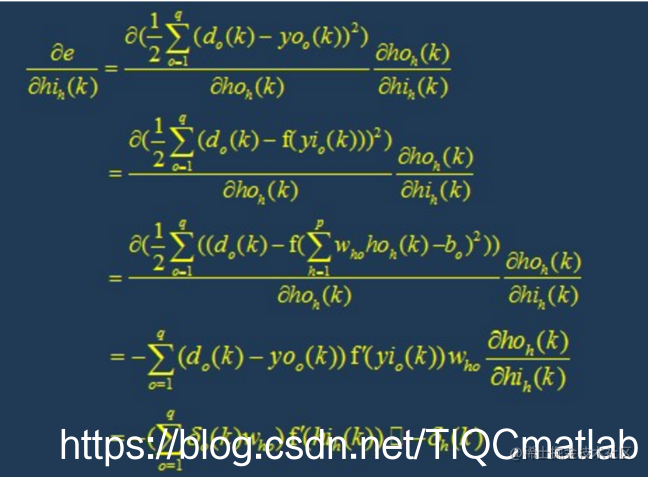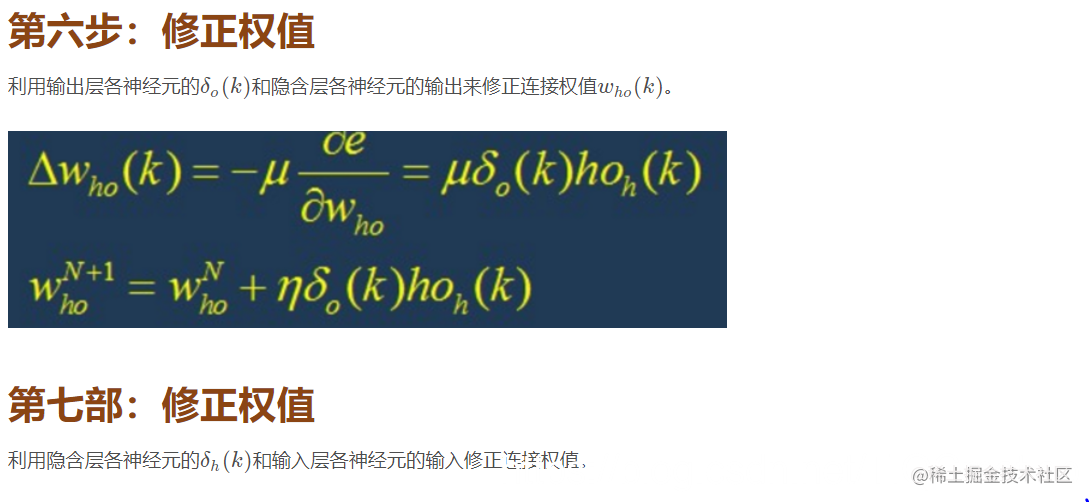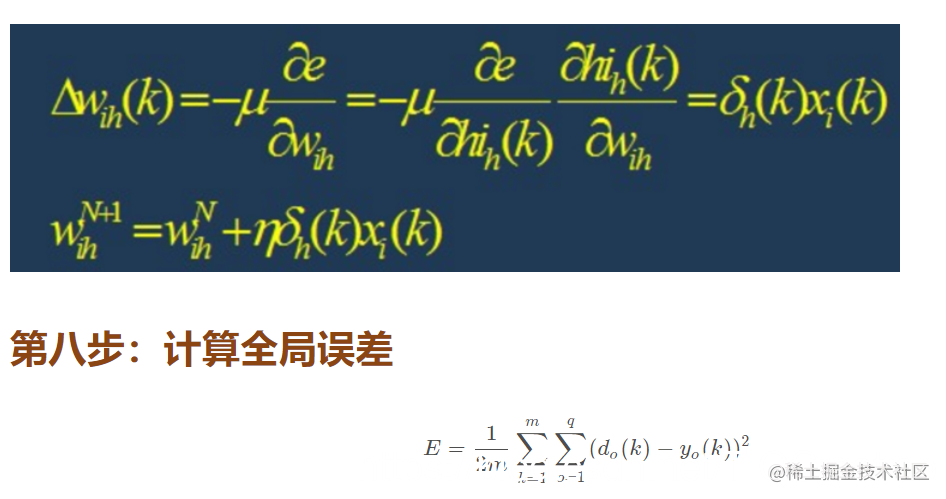6 BP网络的设计

6.1 网络的层数

6.2 隐层神经元的个数

（1）神经元数太少时，网络不能很好的学习，训练迭代的次数也比较多，训练精度也不高。
（2）神经元数太多时，网络的功能越强大，精确度也更高，训练迭代的次数也大，可能会出现过拟合(over fitting)现象。

6.3 初始权值的选取

6.4 学习速率

6.5 期望误差的选取

7 BP网络的局限性
BP网络具有以下的几个问题：

(1)需要较长的训练时间：这主要是由于学习速率太小所造成的，可采用变化的或自适应的学习速率来加以改进。
(2)完全不能训练：这主要表现在网络的麻痹上，通常为了避免这种情况的产生，一是选取较小的初始权值，而是采用较小的学习速率。
(3)局部最小值：这里采用的梯度下降法可能收敛到局部最小值，采用多层网络或较多的神经元，有可能得到更好的结果。

8 BP网络的改进
P算法改进的主要目标是加快训练速度，避免陷入局部极小值等，常见的改进方法有带动量因子算法、自适应学习速率、变化的学习速率以及作用函数后缩法等。 动量因子法的基本思想是在反向传播的基础上，在每一个权值的变化上加上一项正比于前次权值变化的值，并根据反向传播法来产生新的权值变化。而自适应学习 速率的方法则是针对一些特定的问题的。改变学习速率的方法的原则是，若连续几次迭代中，若目标函数对某个权倒数的符号相同，则这个权的学习速率增加， 反之若符号相反则减小它的学习速率。而作用函数后缩法则是将作用函数进行平移，即加上一个常数。

二、源代码

% bp_imageCompress.m
% 基于BP神经网络的图像压缩

%% 清理
clc
clear all
rng(0)

%% 压缩率控制
K=4;
N=2;
row=256;
col=256;

%% 数据输入

% 统一将形状转为row*col
I=imresize(I,[row,col]);

%% 图像块划分，形成K^2*N矩阵
P=block_divide(I,K);

%% 归一化
P=double(P)/255;

%% 建立BP神经网络
net=feedforwardnet(N,'trainlm');
T=P;
net.trainParam.goal=0.001;
net.trainParam.epochs=500;
tic
net=train(net,P,T);
toc

%% 保存结果
com.lw=net.lw{2};
com.b=net.b{2};
[~,len]=size(P); % 训练样本的个数
com.d=zeros(N,len);
for i=1:len
com.d(:,i)=tansig(net.iw{1}*P(:,i)+net.b{1});
end
minlw= min(com.lw(:));
maxlw= max(com.lw(:));
com.lw=(com.lw-minlw)/(maxlw-minlw);
minb= min(com.b(:));
maxb= max(com.b(:));
com.b=(com.b-minb)/(maxb-minb);
maxd=max(com.d(:));
mind=min(com.d(:));
com.d=(com.d-mind)/(maxd-mind);

com.lw=uint8(com.lw*63);
com.b=uint8(com.b*63);
com.d=uint8(com.d*63);
% bp_imageRecon.m

%% 清理
clear,clc
close all

%% 载入数据
col=256;
row=256;
com.lw=double(com.lw)/63;
com.b=double(com.b)/63;
com.d=double(com.d)/63;
com.lw=com.lw*(maxlw-minlw)+minlw;
com.b=com.b*(maxb-minb)+minb;
com.d=com.d*(maxd-mind)+mind;

%% 重建
for i=1:4096
Y(:,i)=com.lw*(com.d(:,i)) +com.b;
end

%% 反归一化
Y=uint8(Y*255);

%% 图像块恢复
I1=re_divide(Y,col,4);

%% 计算性能
fprintf('PSNR :\n  ');
psnr=10*log10(255^2*row*col/sum(sum((I-I1).^2)));
disp(psnr)
a=dir();
for i=1:length(a)
if (strcmp(a(i).name,'comp.mat')==1)
si=a(i).bytes;
break;
end
end

三、运行结果